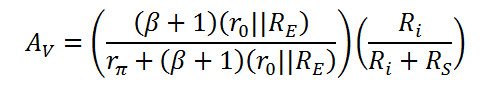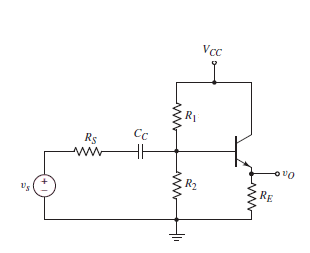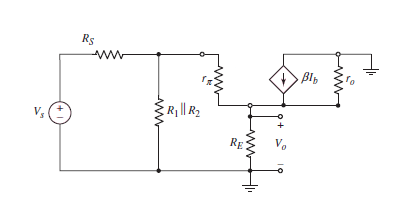# Common Collector Amplifier Calculator (Small Signal Analysis)

This calculator computes the Diffusion Resistance (rp), Small signal Output Resistance (r0) and Output Gain (Av) for a common collector amplifier, i.e. the small signal parameters and output gain of a BJT Amplifier biased using self baising and working in the Common Collector mode. This mode, also known as emitter-follower mode has gain very close to unity and here, output is taken off the emitter. In this configuration the base terminal of the BJT is the input, the emitter is the output and the collector is the common port. This configuration is equivalent to the common drain configuration in a FET transistor.

*Temperature has been asked to calculate the thermal voltage. Please enter T=300K if nothing is given.

V
mA
K-Ohm
K-Ohm
K-Ohm
K

#### Result

K-Ohm
K-Ohm
V/V